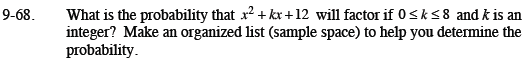Home > CCA2 > Chapter 9 > Lesson 9.2.2 > Problem9-68

9-68.

What is the probability that x2 + kx + 12 will factor if 0 ≤ k ≤ 8 and k is an integer? Make an organized list (sample space) to help you determine the probability. Homework Help ✎How many ways can you multiply to get 12 using the integers from 0 to 8?

How many integers are there from 0 to 8?